### Home > CC1 > Chapter 8 > Lesson 8.1.3 > Problem8-43

8-43.
1. Solve and graph the following inequalities: Homework Help ✎

1. x − 9 < 17

2. x + 12 ≤ 6

3. 10 ≥ 4 + x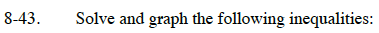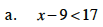Solve the inequalities like an equation. Substitute the inequality symbol with an equal sign.
x − 9 = 17

Isolate the x by adding nine to each side.
x − 9 + 9 = 17 + 9
x = 26

Choose two numbers, one less than 26 and one greater than 26. (Example: 3 and 45) Substitute these numbers for x to decide which direction the arrow should point.
x < 26
45 < 26 (False)
3 < 26 (True)
Since 3 is less than 26, we know that the arrow must point to the left. Every number to the left of 26 on a number scale is less than 26.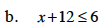Use the same method from part (a).

x ≤ −6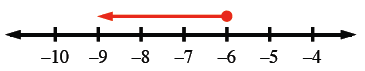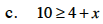Solve using the same method from part (a).